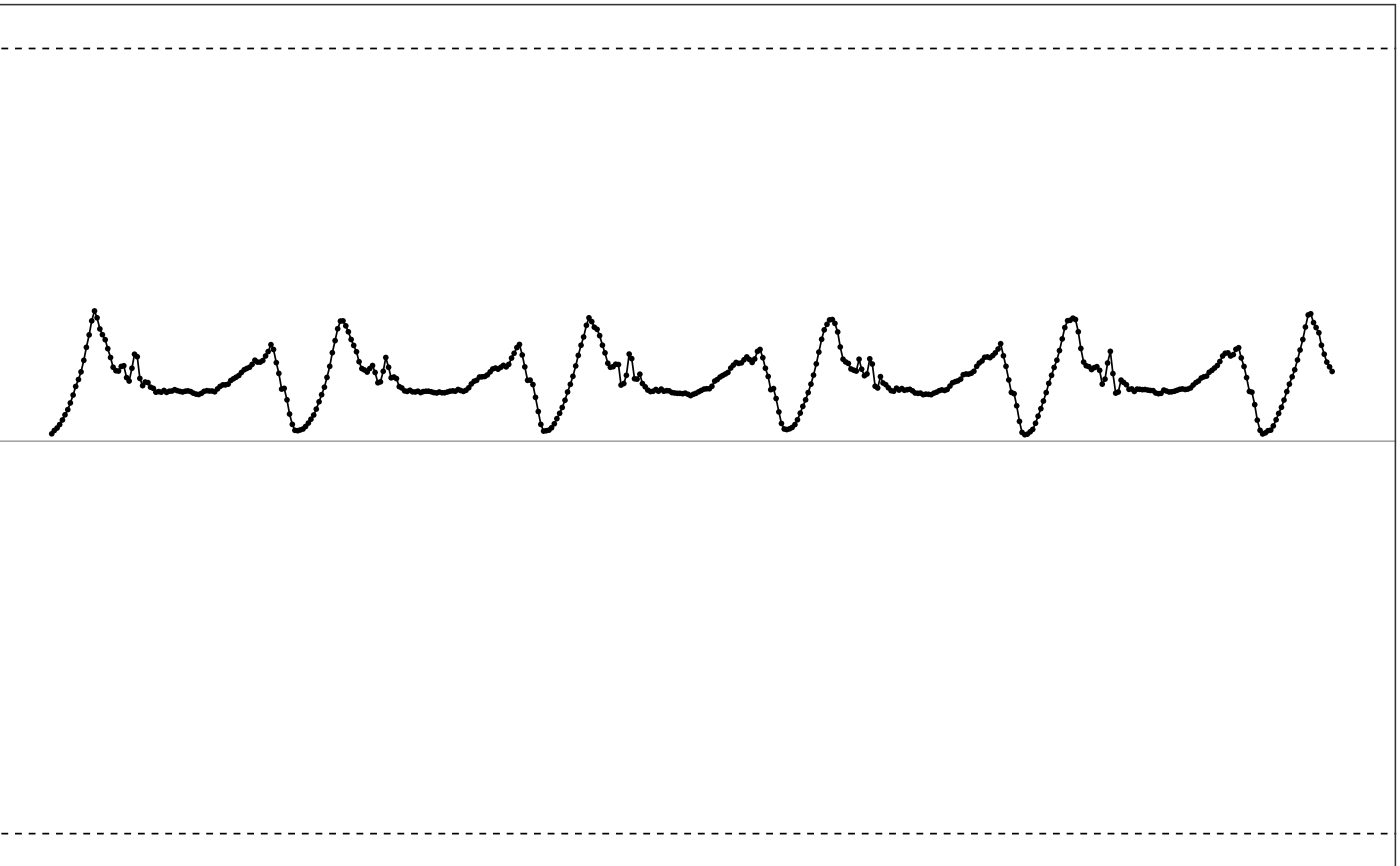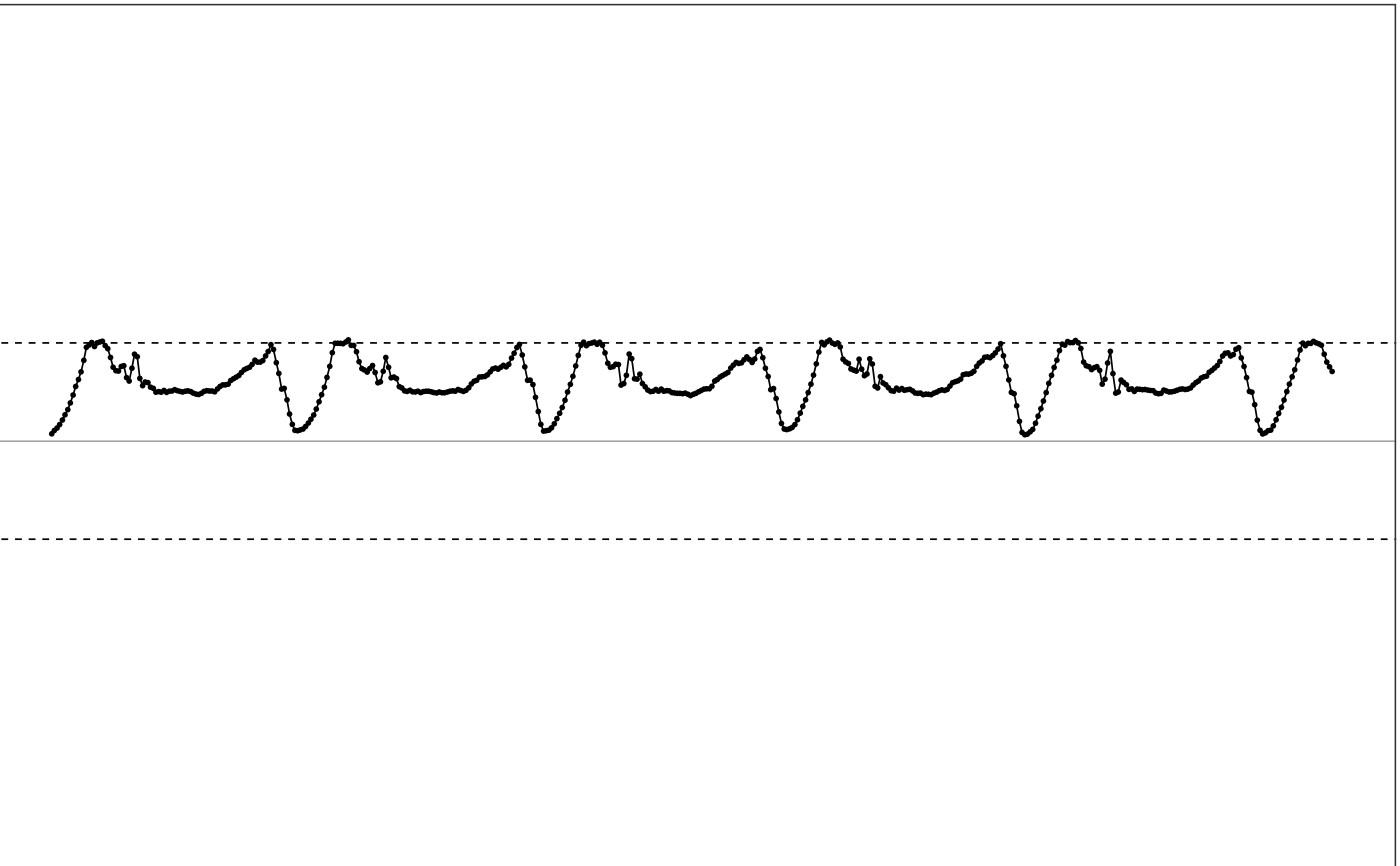cut_off_signal cuts off the input multi-channel accelerometer data according to a new dynamic range, then adds gausian noise to the cut-off samples.

cut_off_signal(df, range = NULL, noise_std = 0.03)

## Arguments

df dataframe. Input multi-channel accelerometer data. numerical vector. The new dynamic ranges to cut off the signal. Should be a 2-element numerical vector. c(low, high), where low is the negative max value the device can reach and high is the positive max value the device can reach. Default is NULL, meaning the function will do nothing but return the input data. number. The standard deviation of the added gaussian noise.

## Value

dataframe. The multi-channel accelerometer data with the new dynamic range as specified in range.

## Details

This function simulates the behavior that a low dynamic range device is trying to record high intensity movement, where recorded accelerometer signal will be cut off at the dynamic range, but the true movement should have higher acceleration values than the dynamic range. This function also adds gaussian noise to the cut off samples to better simulate the real world situation.

## How is it used in MIMS-unit algorithm?

This function is a utility function that is used to simulate the behaviors of low dynamic range devices during algorithm validation.

Other utility functions: clip_data(), interpolate_signal(), parse_epoch_string(), sampling_rate(), segment_data(), simulate_new_data()

## Examples

  # Use sample data for testing
df = sample_raw_accel_data

# Show df
illustrate_signal(df, range=c(-8, 8))# cut off the signal to c(-2, 2)
new_df = cut_off_signal(df, range=c(-2, 2), noise_std=0.03)

# Show new df
illustrate_signal(new_df, range=c(-2, 2))